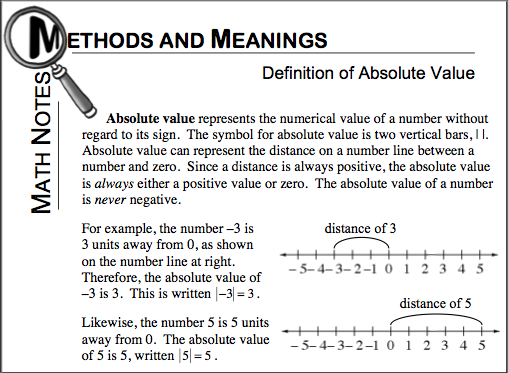### Home > CCAA8 > Chapter 1 Unit 1 > Lesson CCA: 1.1.1 > Problem1-5

1-5.

Evaluate each absolute value expression. Review the Math Notes box in the lesson for the definition of absolute value.

Math Notes box from this lesson below for help with this problem.

1. $| 54 |$

$54$

1. $-|-7\frac{3}{5}|$

Since the absolute value of a number is always positive, take the opposite of the number.

$-7\frac{3}{5}$

1. $| 3 | - | - 1 |$

Find the absolute value of the two numbers, then do the subtraction.

1. $| 2.2 - 5.13 |$

This is the opposite of part (c), do the subtraction first, then find the absolute value of the answer.

$2.93$